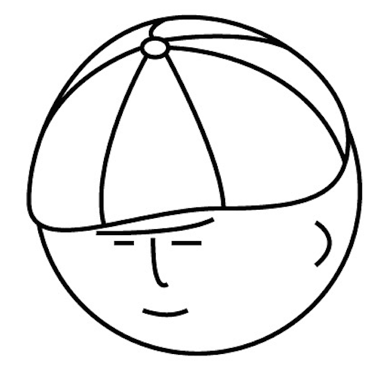マツシタのお勉強メモ

マツシタのお勉強

welcome to my engineering blog
Hacker RankMaximum Size Subarray Sum Equals k

Problem discuss.leetcode.com Solution This problem can be solved by using HashMap effectively. First, we store each sum of range from 0 to i (0 <= i < N). While we traverse the array, we check if the (sum - k) is stored in HashMap. If the …